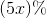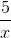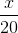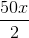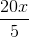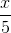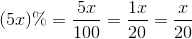## Example Questions

### Example Question #1 : How To Find A Fraction From A Percentage

We asked 1000 people their preferences of movie genres. 7.5% of those asked preferred horror movies, 35% preferred romantic films, 20% preferred dramas and 37.5% preferred comedies. How many people liked horror films?

35

7.5

75

750

37.5

75

Explanation:

People who prefer horror films = 1000 people x 7.5% = 1000 x 0.075 = 75 people

### Example Question #2 : How To Find A Fraction From A Percentage

There are 20 students in a class. 35 percent of the students in the class are boys. What fraction of the class is girls?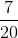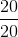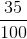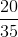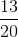Explanation:

There are 20 total students in the class. 35% of 20 is 7. There are 7 boys in the class.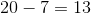girls in the class.

### Example Question #3 : How To Find A Fraction From A Percentage

Which of the following represents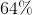as a fraction?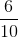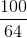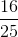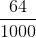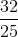Explanation:

To represent a percentage as a fraction, keep the percentage in the numerator and putin the denominator.

Therefore: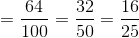### Example Question #4 : How To Find A Fraction From A Percentage

Which of the following fractions is equivalent to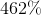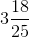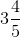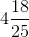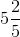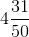Explanation:

To convert an integer percentage into an improper fraction, set the percentage as a fraction overand simplify as far as you can. At that point, simply convert the improper fraction into a mixed fraction.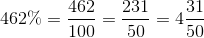### Example Question #5 : How To Find A Fraction From A Percentage

A politician promises to spend up to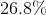of his budget on improving roads. Which of the following is closest to the fraction of their budget the politician must devote to road improvement?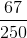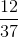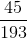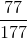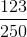Explanation:

To convert a decimal percentage into a fraction, first convert it to a decimal: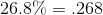Now, set ths as a fraction with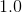as the denominator (expanding to as many significant digits as you need to line the fraction up):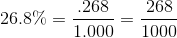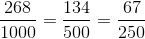### Example Question #6 : How To Find A Fraction From A Percentage

Convert to a fraction: